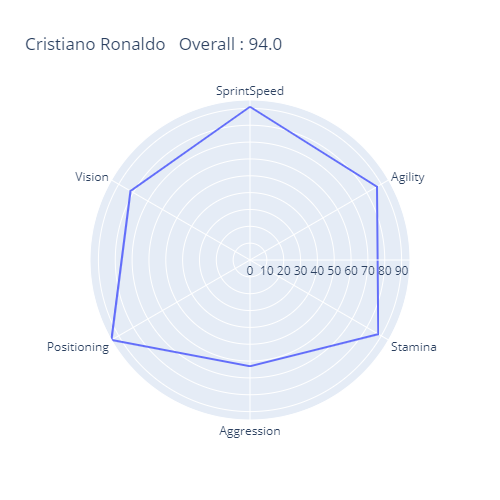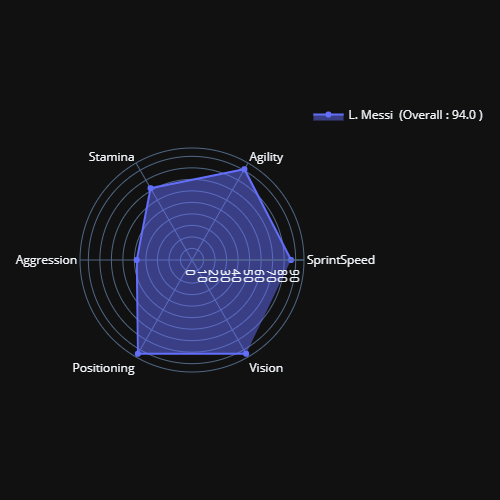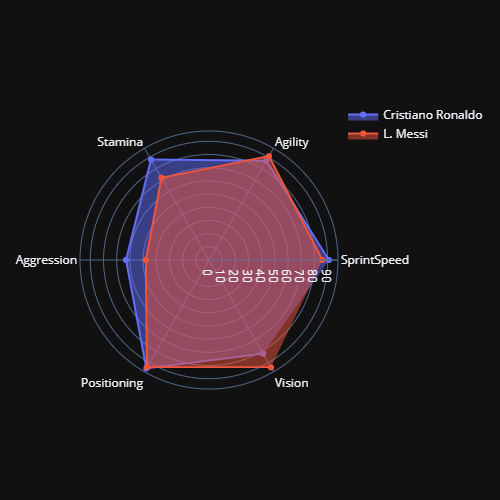Open in App
Not now

# Star Charts in Python

• Last Updated : 09 Mar, 2021

Star plot or Radar Chart is used for displaying multivariate data, in which each variable provides the measure of some common property of each observation. Each star in the plot represents a single observation. Star plot is used to examine the relative values of a single data point and locate similar and dissimilar points.

The star plot consists of the sequence of equiangular spokes called radii with each spoke representing the value of observation on one of the variables. The data length of a spoke is proportional to the magnitude of the variable for the point related to the maximum data point in that variable. A line is drawn connecting to all the data points

The star plot can be used to answer the following questions:

• which variable is dominant for a given observation?
• which observations are most similar i.e are there any clusters of observations?
• are there outliers?

### Applications

• Star plot is generally used in sports visualizations and games such as FIFA, Pro Evolution Soccer (PES) to visualize the player’s strengths and weaknesses.
• Star plot can also be used for quality improvement in order to visualize the different performance metrics.

### Limitations:

• Star plot is not a good way to visualize the ordinal or categorical variable which does not represent one category is better or worse than the other.
• Star plot sometimes create a connection between two unrelated variables. That’s why a star plot cannot be trusted for making trade-off decisions.
• The ordering of variables also affects the shape of the star plot. For Example, the values 9, 2, 9, 1, 9, 1 produce a different spike than 9, 9, 9, 2, 1, 1.

### Implementation:

• For this implementation, we will be using plotly and some general package. These packages are pre-installed in colaboratory but can be installed in the local environment. To install the plotly in the local environment, follow these instructions.
• For this implementation, we will be using the FIFA-19 players dataset, you can obtain the dataset from Kaggle.

## Python3

 `# code``import` `pandas as pd``import` `numpy as np``import` `plotly.express as px``import` `plotly.graph_objects as go`` ` `# read dataset from csv and perform preprocessing``data ``=` `pd.read_csv(``'data.csv'``)``data.head()`` ` `# remove goalkeepers data for performing fair comparison``goalkeeper_index ``=` `data[data[``'Position'``] ``=``=` `'GK'` `].index``data.drop(index_names,inplace``=``True``)`` ` `# remove columns which are not required for this visualisation purpose``plt_vars ``=` `[``'SprintSpeed'``,``'Agility'``,``'Stamina'``,``'Aggression'``,``            ``'Positioning'``,``'Vision'``]``data.drop(data_cols, axis ``=``1``, inplace``=``True``)`` ` `# plot unfilled scatter plot``px.line_polar(data, r ``=` `pd.Series(data.loc[``1``,plt_vars].values),``              ``theta``=``plt_vars,line_close``=``True``,``              ``title ``=``  ``f'{data.loc[``1``,``"Name"``]} \n Overall : {data.loc[``1``,``"Overall"``]} \``          ``Potential: {data.loc[``1``,``"Potential"``]}', width ``=` `500``)`` ` `# plot filled star plot``fig ``=` `go.Figure(data``=``go.Scatterpolar(``  ``r``=``pd.Series(data.loc[``0``,plt_vars].values),``  ``theta``=``plt_vars,``  ``fill``=``'toself'``,``  ``name ``=``f'{data.loc[``0``,``"Name"``]}  (Overall : {data.loc[``0``,``"Overall"``]}  \``        ``Potential: {data.loc[``0``,``"Potential"``]} )'),)`` ` `fig.update_layout(``  ``polar``=``dict``(``    ``radialaxis``=``dict``(``      ``visible``=``True``,``    ``),``  ``),``  ``template ``=` `'plotly_dark'``,``  ``showlegend``=``True``,``   ` `)`` ` `fig.show()`` ` `# plot star plot for comparison``fig ``=` `go.Figure()`` ` `fig.add_trace(go.Scatterpolar(``      ``r``=``pd.Series(data.loc[``1``,plt_vars].values),``      ``theta``=``plt_vars,``      ``fill``=``'toself'``,``      ``name``=``data.loc[``1``, ``'Name'``]``))``fig.add_trace(go.Scatterpolar(``      ``r``=``pd.Series(data.loc[``0``,plt_vars].values),``      ``theta``=``plt_vars,``      ``fill``=``'toself'``,``      ``name``=``data.loc[``0``, ``'Name'``]``))`` ` `fig.update_layout(``  ``polar``=``dict``(``    ``radialaxis``=``dict``(``      ``visible``=``True``          ``)),``    ``template ``=` `'plotly_dark'``,`` ` `  ``showlegend``=``True``)`` ` `fig.show()`unfilled Star plotFilled Star plotComparison with Star plot

### References:

My Personal Notes arrow_drop_up